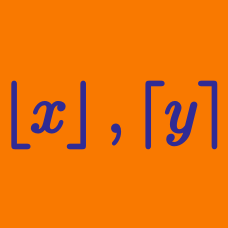Algebra

# Floor and Ceiling Functions: Level 4 Challenges

$\large \sum_{k=1}^{202} \left \lceil \sqrt k \ \right \rceil = \ ?$

Find the positive integer $n$, for which $\lfloor \log_2{1}\rfloor+\lfloor\log_2{2}\rfloor+\lfloor\log_2{3}\rfloor+\cdots+\lfloor\log_2{n}\rfloor=1994.$

What is the minimum integer value of $x$ that satisfies the equation

$\lfloor{\sqrt{x}}\rfloor - \lfloor{\sqrt{x+34}}\rfloor=0 ?$

This problem is posed by Siam H.

Details and assumptions

The function $\lfloor x \rfloor: \mathbb{R} \rightarrow \mathbb{Z}$ refers to the greatest integer smaller than or equal to $x$. For example $\lfloor 2.3 \rfloor = 2$ and $\lfloor -5 \rfloor = -5$.

$\left\lfloor x+0.19 \right\rfloor +\left\lfloor x+0.20 \right\rfloor +\left\lfloor x+0.21 \right\rfloor + \ldots + \left\lfloor x+0.91 \right\rfloor =542$

If $x$ satisfies the equation above, find the value of $\left\lfloor 100x \right\rfloor$.

Note that $\left\lfloor X \right\rfloor$ denote the floor function of $X$.

The number of real solutions of $7\lfloor x\rfloor + 23\{x\}=191$ is?

×